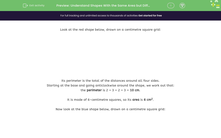# Understand Shapes With the Same Area but Different Perimeter

In this worksheet, students will be asked to use their knowledge of how to calculate the area and perimeter of shapes. They will have an understanding that shapes with the same area can have different perimeters and vice versa. This worksheet will involve problem solving using these concepts.Key stage:  KS 2

Curriculum topic:   Measurement

Curriculum subtopic:   Calculate Areas

Popular topics:   Area worksheets

Difficulty level:#### Worksheet Overview

Look at the red shape below, drawn on a centimetre square grid:

Its perimeter is the total of the distances around all four sides.

Starting at the base and going anticlockwise around the shape, we work out that:

the perimeter is 2 + 3 + 2 + 3 = 10 cm.

It is made of 6-centimetre squares, so its area is 6 cm2.

Now look at the blue shape below, drawn on a centimetre square grid:

Its perimeter is the total of the distances around all its sides.

Starting at the base and going anticlockwise around the shape, we work out that:

the perimeter is 1 + 1 + 1 + 2 + 1 + 1 + 1 + 2 = 10 cm.

This is the same perimeter as the red shape above.

But it is only made of 4-centimetre squares, so its area is only 4 cm2.

Shapes with the same perimeter can have different areas.

Shapes with the same area can have different perimeters.

You will need to use this knowledge to solve a series of questions and solve some problems.

### What is EdPlace?

We're your National Curriculum aligned online education content provider helping each child succeed in English, maths and science from year 1 to GCSE. With an EdPlace account you’ll be able to track and measure progress, helping each child achieve their best. We build confidence and attainment by personalising each child’s learning at a level that suits them.

Get started